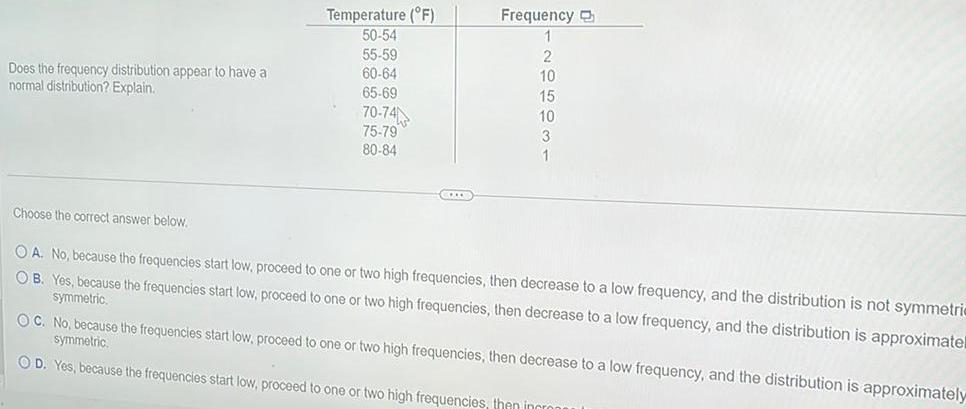Question:

# Does the frequency distribution appear to have a normal

Last updated: 9/19/2023Does the frequency distribution appear to have a normal distribution Explain Temperature F 50 54 55 59 60 64 65 69 70 74 75 79 80 84 C Frequency 1 2 10 15 10 3 1 Choose the correct answer below OA No because the frequencies start low proceed to one or two high frequencies then decrease to a low frequency and the distribution is not symmetric OB Yes because the frequencies start low proceed to one or two high frequencies then decrease to a low frequency and the distribution is approximatel symmetric OC No because the frequencies start low proceed to one or two high frequencies then decrease to a low frequency and the distribution is approximately symmetric OD Yes because the frequencies start low proceed to one or two high frequencies then incron